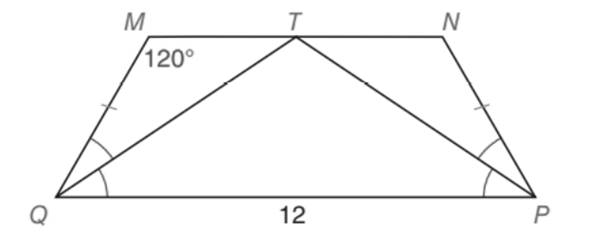Chapter 5.5, Problem 34EElementary Geometry For College St...

7th Edition
Alexander + 2 others
ISBN: 9781337614085

Solutions

Chapter
SectionElementary Geometry For College St...

7th Edition
Alexander + 2 others
ISBN: 9781337614085
Textbook Problem

Note: Exercises preceded by an asterisk are of a more challenging nature. Given: Isosceles trapezoid M N P Q with Q P = 12 and m ∠ M = 120 ° ; the bisectors of ∠ s M Q P and Find: The perimeter of M N P QTo determine

To find:

The perimeter of the isosceles trapezoid MNPQ with QP=12 and mM=120°; the bisecfors of SMQP and NPQ meet at point T on MN¯.

Explanation

Definition:

The perimeter of any closed figure is given by the sum of all the lengths of its boundary.

Calculation:

Given,

An isosceles trapezoid MNPQ with QP=12

mM=120°

The bisectors of MQP and NQP meet at point T on MN¯

In the above given isosceles trapezoid, we have the following congruent measurement.

A pair of the non-parallel sides of any isosceles trapezoid is congruent.

MQ¯NP¯

Each pair of base angles are congruent.

mQMNmPNM

Thus,

mQMN=120°=mPNM

Also

mMQPmNPQ

Since, the above congruent angles mMQP and mNPQ are bisected by the line segments QT¯ and PT¯, all the four angles formed by bisector are congruent.

mMQTmTQPmNPTmTPQ

In a trapezoid sum of the adjacent angles which lie on the opposite bases is 180°

mMQP+mQMN=180°

mMQP+120°=180°

Add; -120° on both sides,

mMQP+120°+-120°=180°-120°

mMQP+120+-120=180°-120°

mMQP=60°

Thus, mMQP=mNPQ=60°

Also,

mMQT=mTQP=mNPT=mTPQ=12·mMQP (or) 12·mNPQ

mMQT=mTQP=mNPT=mTPQ=30°

By the property that the sum of all the three inferior angle of any triangle is 180°, we have

In PQT

mP=30°

mQ=30° and

Thus,

mT=120°

Also, in MTQ

mM=120°

mQ=30°, and

Thus,

mT=30°

Further, in TNP

mP=30°,

mN=120°, and

Thus,

mT=30°

Now consider the TPQ and draw TS¯PQ.

Since, TPQ is an isosceles triangle with sides TQ¯ and TP¯ congruent, the line segment TS¯ bisects the angle T of the TPQ and also the side PQ¯.

Thus, we have two right triangle TSQ and TSP of 30°-60°-90° type.

In TSQ

TQ= Hypotenuse (Opposite to 90°)

QS= Linger leg (Opposite to 60°)

TS= shorter leg = 6 (Opposite to 30°)

By the application of 30-60-90 theorem

30°-60°-90° theorem

Still sussing out bartleby?

Check out a sample textbook solution.

See a sample solution

The Solution to Your Study Problems

Bartleby provides explanations to thousands of textbook problems written by our experts, many with advanced degrees!

Get Started

In Exercises 69-74, rationalize the numerator. 71. 2yx

Applied Calculus for the Managerial, Life, and Social Sciences: A Brief Approach

Sketch the graphs of the equations in Exercises 512. xy=x2+1

Finite Mathematics and Applied Calculus (MindTap Course List)

Evaluate the integral. 81. 1sinxdx

Calculus: Early Transcendentals

, where E is the wedge-shaped solid shown at the right, equals:

Study Guide for Stewart's Multivariable Calculus, 8th